# 活用字符串

## 宏

 A B 1 [+,-,*,/] =A1(rand(4)+1) 2 =rand(1000) =rand(1000) 3 =A2\${B1}B2

B1A1的操作符列表中，随机获取一种四则运算符号，A2B2随机生成两个1000以内的整数，在A3中计算四则运算的结果。

B1A2B2中的结果如下：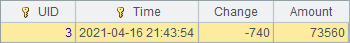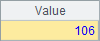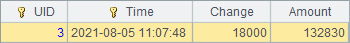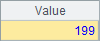A B 1 A"+"A " 2 ="aabb\${A1}ccdd" 3 =\${B1}aabb\${A1}ccdd"

A2中的\${A1}没有作用，最终A2中的结果就是引号中的字符串：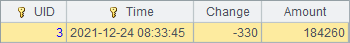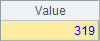A B 1 4779736 AL 2 710231 AK 3 6392017 AZ 4 2915918 AR 5 37253956 CA 6 =[A1:A5].pselect(~>5000000) 7 =B\${string(A6)}

A6中，找到第一个人口大于5,000,000的数据所在的行；在A7的表达式中，根据这个行号取出B列中对应的单元格名称，从而获得该州的缩写。需要注意，宏中的表达式必须返回一个字符串，因此在A7中用宏生成单元格名称时，需要用string() 函数将A6中获得的行号转换为字符串。A6A7中的结果如下：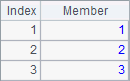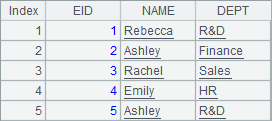A B 1 4779736 AL 2 710231 AK 3 6392017 AZ 4 2915918 AR 5 37253956 CA 6 =[A1:A5].pselect@a(~>5000000) 7 =A6.(B\${string(A6.~)})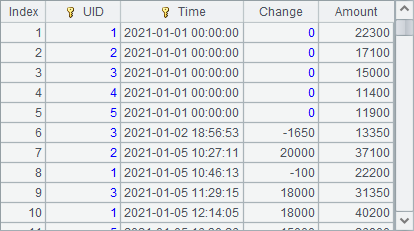A7中，根据A6中的序列循环，试图每次取出对应州的简称，结果如下：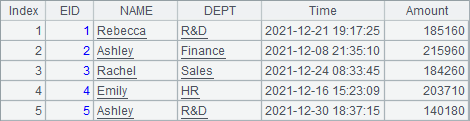## eval 函数

 A B 1 [+,-,*,/] =A1(rand(4)+1) 2 =rand(1000) =rand(1000) 3 =eval("A2"+B1+"B2")

B1A2B2中的结果如下：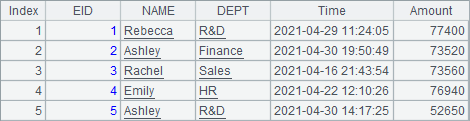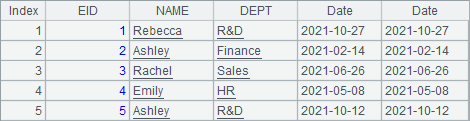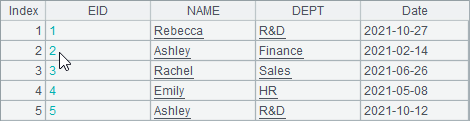A3中可以看到eval()函数与前面使用宏时的不同，此时A3中即为计算893+466的结果：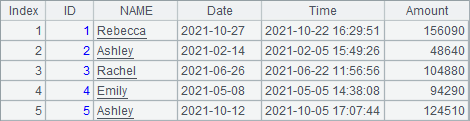eval函数的功能比宏更为强大，在生成的表达式中使用参数时，可以实现类似自定义函数的功能，如：

 A 1 =eval("?.count(~>5)",[2,3,4,5,6,7,8]) 2 =eval("if (?1>0 && ?2>0 && ?3>0 && ?1+?2>?3 && ?1+?3>?2 && ?2+?3>?1)",3,2,5)

A1中，用eval函数计算一个系列中大于5的成员个数，eval生成的表达式中?代表使用的参数。A2中判断3个参数能否构成一个三角形的三边长，其中?1?2?3分别代表第1个，第2个和第3个参数。A1A2中的计算结果如下：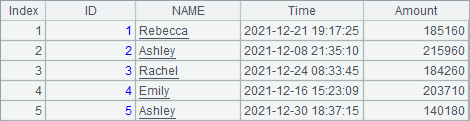A B 1 4779736 AL 2 710231 AK 3 6392017 AZ 4 2915918 AR 5 37253956 CA 6 =[A1:A5].pselect@a(~>5000000) 7 =A6.(eval("B"+string(A6.~)))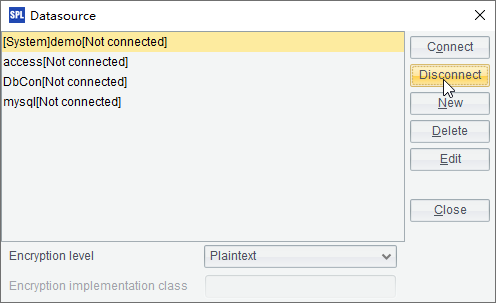## 字符串常数

 A 1 ="abc"+\$[ABC] 2 ="\"[abc]\""+\$["[ABC\]"]

A1A2中的计算结果如下：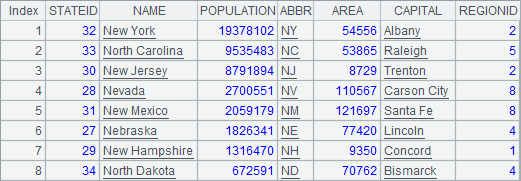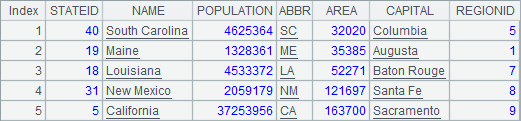A B 1 select * from STATES =demo.query(A1) 2 POPULATION>10000000 =demo.query(\$[\${A1} where \${A2}]) 3 left(ABBR,1)='C' =demo.query(\$[\${A1} where \${A2} and \${A3}])

B2B3中，在字符串常数中，嵌套使用了宏，用单元格值生成query函数的查询字符串。其中，B2中查询人口大于10,000,000的州：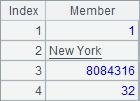B3中查询人口大于10,000,000且缩写的首字母为C的州：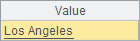A B 1 select * from STATES =demo.query(A1) 2 3 POPULATION>10000000 =demo.query(\$[\${A1} where \${A3]) 4 left(ABBR,1)='C' =demo.query(\$[\${A1} where \${A3} and \${A4}])